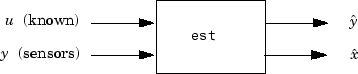Main Content

# estim

Form state estimator given estimator gain

## Syntax

```est = estim(sys,L) est = estim(sys,L,sensors,known) ```

## Description

`est = estim(sys,L) ` produces a state/output estimator `est` given the plant state-space model `sys` and the estimator gain `L`. All inputs w of `sys` are assumed stochastic (process and/or measurement noise), and all outputs y are measured. The estimator `est` is returned in state-space form (SS object).

For a continuous-time plant `sys` with equations

`$\begin{array}{l}\stackrel{˙}{x}=Ax+Bw\\ y=Cx+Dw\end{array}$`

`estim` uses the following equations to generate a plant output estimate $\stackrel{^}{y}$ and a state estimate $\stackrel{^}{x}$, which are estimates of y(t)=C and x(t), respectively:

`$\begin{array}{l}\stackrel{˙}{\stackrel{^}{x}}=A\stackrel{^}{x}+L\left(y-C\stackrel{^}{x}\right)\\ \left[\begin{array}{c}\stackrel{^}{y}\\ \stackrel{^}{x}\end{array}\right]=\left[\begin{array}{c}C\\ I\end{array}\right]\stackrel{^}{x}\end{array}$`

For a discrete-time plant `sys` with the following equations:

`$\begin{array}{l}x\left[n+1\right]=Ax\left[n\right]+Bw\left[n\right]\\ y\left[n\right]=Cx\left[n\right]+Dw\left[n\right]\end{array}$`

`estim` uses estimator equations similar to those for continuous-time to generate a plant output estimate $y\left[n|n-1\right]$ and a state estimate $x\left[n|n-1\right]$, which are estimates of y[n] and x[n], respectively. These estimates are based on past measurements up to y[n-1].

`est = estim(sys,L,sensors,known) ` handles more general plants `sys` with both known (deterministic) inputs u and stochastic inputs w, and both measured outputs y and nonmeasured outputs z.

`$\begin{array}{l}\stackrel{˙}{x}=Ax+{B}_{1}w+{B}_{2}u\\ \left[\begin{array}{c}z\\ y\end{array}\right]=\left[\begin{array}{c}{C}_{1}\\ {C}_{2}\end{array}\right]x+\left[\begin{array}{c}{D}_{11}\\ {D}_{21}\end{array}\right]w+\left[\begin{array}{c}{D}_{12}\\ {D}_{22}\end{array}\right]u\end{array}$`

The index vectors `sensors` and `known` specify which outputs of `sys` are measured (y), and which inputs of `sys` are known (u). The resulting estimator `est`, found using the following equations, uses both u and y to produce the output and state estimates.

`$\begin{array}{l}\stackrel{˙}{\stackrel{^}{x}}=A\stackrel{^}{x}+{B}_{2}u+L\left(y-{C}_{2}\stackrel{^}{x}-{D}_{22}u\right)\\ \left[\begin{array}{c}\stackrel{^}{y}\\ \stackrel{^}{x}\end{array}\right]=\left[\begin{array}{c}{C}_{2}\\ I\end{array}\right]\stackrel{^}{x}+\left[\begin{array}{c}{D}_{22}\\ 0\end{array}\right]u\end{array}$`
``

## Examples

Consider a state-space model `sys` with seven outputs and four inputs. Suppose you designed a Kalman gain matrix L using outputs 4, 7, and 1 of the plant as sensor measurements and inputs 1, 4, and 3 of the plant as known (deterministic) inputs. You can then form the Kalman estimator by

```sensors = [4,7,1]; known = [1,4,3]; est = estim(sys,L,sensors,known) ```

See the function `kalman` for direct Kalman estimator design.

## Tips

You can use the functions `place` (pole placement) or `kalman` (Kalman filtering) to design an adequate estimator gain L. Note that the estimator poles (eigenvalues of A-LC) should be faster than the plant dynamics (eigenvalues of A) to ensure accurate estimation.

## See Also

| | | | | | (System Identification Toolbox) | (System Identification Toolbox)

Introduced before R2006a

## Support

#### Learn how to automatically tune PID controller gains

Download code examples